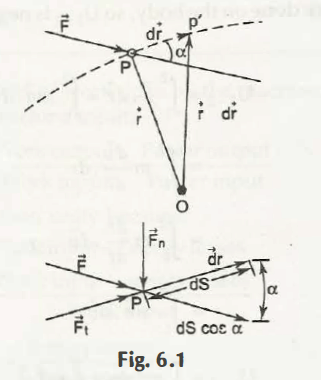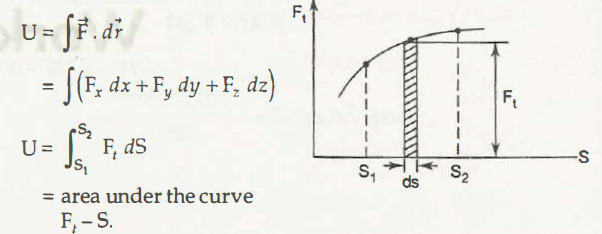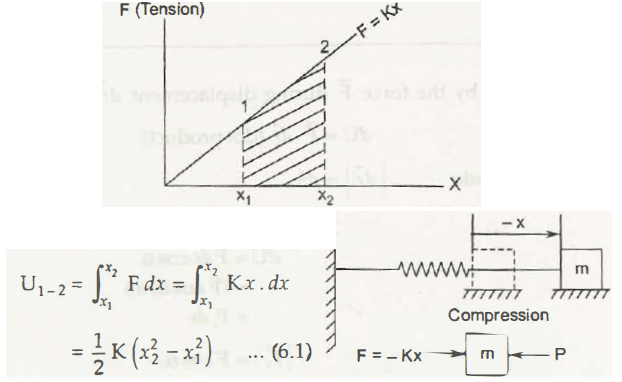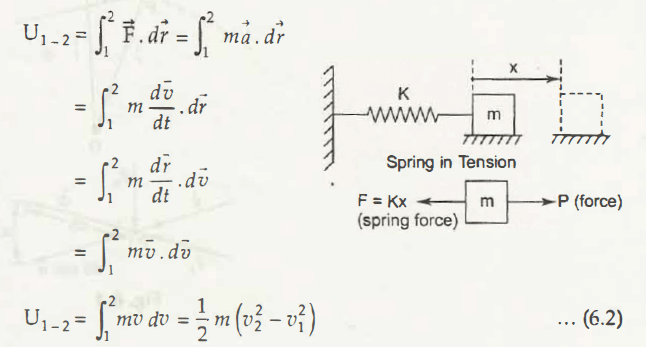Work can be defined as the application of force to produce a displacement in the direction of force. It is a scalar product.

Mathematically,

dU =   .

or,

|| = dSFrom Fig. 6.1, the above equation can be written as,

dU = F dS cos

= (F cos ) dS

= Ft dS                                             (|t|= F cos )

Note:

From the above equation, work can also be defined as product of force in the direction of its application and displacement in the same direction. In other words, work is the product of component of force and its displacement.

See Fig. 6.1, dS and F is at right angle (900). Thus, force Fn will be zero as it does no work. Consider the component of force Ft, the value of work done will be positive if Ft will act on the same direction of displacement.

Similarly, the value of work done will be negative if Ft will act on the opposite direction of displacement.

Unit of Work:

S.I. unit of work is Joule (J) or Newton-meter (N-m). For the force and displacement calculation, we use the unit of work as N-m. (Joule will be used in terms of energy)

1. Calculating the magnitude of force when its application is shown using the figure below:U =

= x dx + Fydy + Fz dz)

= t dS

= Ft – S

Where,

Ft – S = Area under the curve

1. Calculate the magnitude of force:To calculate the force, it can be written as,

U1 – 2     =

=

= ½ K (x22 – x12)

Note:

Since the work is done on the body, its sign will be negative.

Again,

Calculate the magnitude of force as shown in figure belowU1 – 2     =

=

=

=

=

Hence,

Work done will be,

U1 – 2     = ½ m (v22 – v12)

Links of Next Mechanical Engineering Topics:-### Customer Reviews

My Homework Help
Rated 5.0 out of 5 based on 510 customer reviews at